# java中Excel行列操作，POI对Word的基本操作有哪些？

BSO 2020-09-11 10:04:56 java常见问答 6557

```HSSFSheet sheet = workbook.createSheet("Test0"); // 创建工作表(Sheet)
sheet.groupRow(1, 3); //组合行
sheet.groupRow(2, 4); //组合行
sheet.groupColumn(2, 7); //组合列```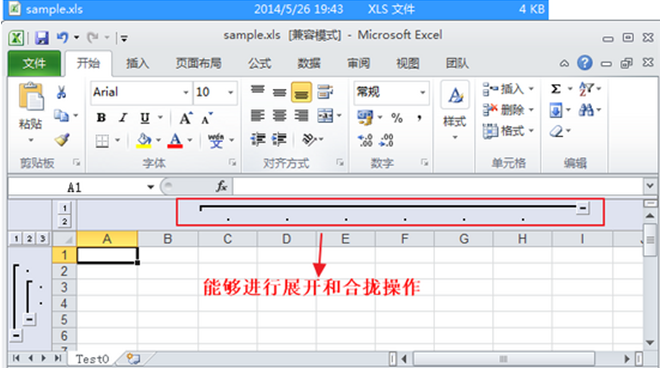```HSSFSheet sheet = workbook.createSheet("Test0"); // 创建工作表(Sheet)
sheet.createFreezePane(2, 3, 15, 25); //冻结行列```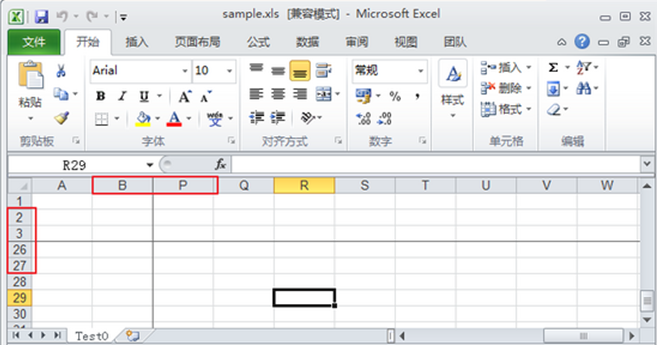1.第一个参数表示要冻结的列数;

2.第二个参数表示要冻结的行数，这里只冻结列所以为0;

3.第三个参数表示右边区域可见的首列序号，从1开始计算;

4.第四个参数表示下边区域可见的首行序号，也是从1开始计算，这里是冻结列，所以为0;

```FileInputStream stream = new FileInputStream(filePath);
HSSFWorkbook workbook = new HSSFWorkbook(stream);
HSSFSheet sheet = workbook.getSheet("Test0");
sheet.shiftRows(2, 4, 2); //把第3行到第4行向下移动两行```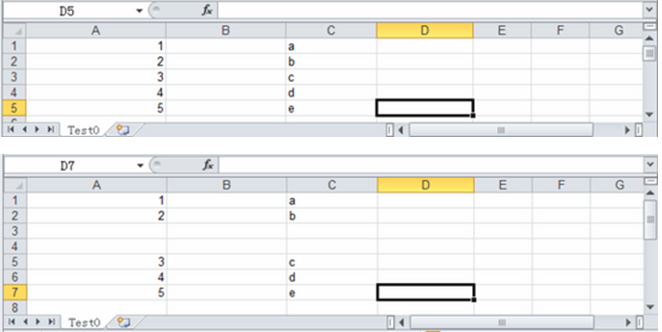1.startRow：需要移动的起始行;

2.endRow：需要移动的结束行;

3.n：移动的位置，正数表示向下移动，负数表示向上移动;

```XWPFDocument doc = new XWPFDocument(); // 创建Word文件
XWPFParagraph p = doc.createParagraph(); // 新建一个段落
p.setAlignment(ParagraphAlignment.CENTER); // 设置段落的对齐方式
p.setBorderBottom(Borders.DOUBLE); //设置下边框
p.setBorderTop(Borders.DOUBLE); //设置上边框
p.setBorderRight(Borders.DOUBLE); //设置右边框
p.setBorderLeft(Borders.DOUBLE); //设置左边框
XWPFRun r = p.createRun(); //创建段落文本
r.setText("POI创建的Word段落文本");
r.setBold(true); //设置为粗体
r.setColor("FF0000"); //设置颜色
p = doc.createParagraph(); // 新建一个段落
r = p.createRun();
r.setText("POI读写Excel功能强大、操作简单。");
XWPFTable table = doc.createTable(3, 3); //创建一个表格
table.getRow(0)
.getCell(0)
.setText("表格1");
table.getRow(1)
.getCell(1)
.setText("表格2");
table.getRow(2)
.getCell(2)
.setText("表格3");
FileOutputStream out = newFileOutputStream("d:\\POI\\sample.doc");
doc.write(out);
out.close();```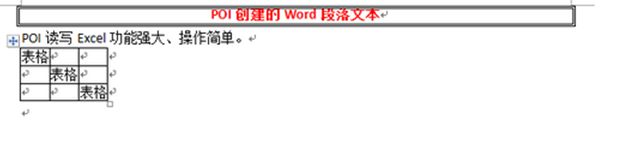```FileInputStream stream = newFileInputStream("d:\\POI\\sample.doc");
XWPFDocument doc = new XWPFDocument(stream); // 创建Word文件
for (XWPFParagraph p: doc.getParagraphs()) //遍历段落
{
System.out.print(p.getParagraphText());
}
for (XWPFTable table: doc.getTables()) //遍历表格
{
for (XWPFTableRow row: table.getRows())
{
for (XWPFTableCell cell: row.getTableCells())
{
System.out.print(cell.getText());
}
}
}```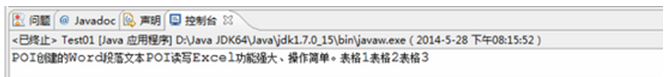java中Excel公式的计算和函数，实例展示

java中Excel单元格对齐包含什么?使用边框怎么做?

java中Excel字体的设置，背景和纹理的操作